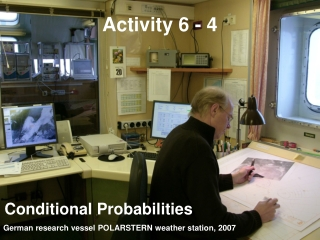DownloadDownload PresentationActivity 6 - 4

# Activity 6 - 4

Télécharger la présentation## Activity 6 - 4

- - - - - - - - - - - - - - - - - - - - - - - - - - - E N D - - - - - - - - - - - - - - - - - - - - - - - - - - -
##### Presentation Transcript

1. Activity 6 - 4 Conditional Probabilities German research vessel POLARSTERN weather station, 2007

2. Objectives • Identify a conditional probability problem • Determine conditional probabilities using sample space or the data from a table • Determine conditional probabilities using a formula

3. Vocabulary • None new

4. Activity It has rained for the past three days. However, the weather forecast for tomorrow’s softball game is sunny and no chance of rain. You decide not to take a rain jacket, only to get drenched when there is a sudden downpour during the game. Although not perfect, the level of accuracy of weather forecasting has increased significantly through the use of computer models. These models analyze current data and predict atmospheric conditions at some short period of time form that moment. Based on these predictions, another set of atmospheric conditions are predicted. This process continues until the forecast for the day and the extended forecast for the next several days are completed.

5. Activity cont What does the level of accuracy in the weather forecasting model described above depend upon? The level of accuracy decreases as the forecasts extend several days ahead. Explain. The accuracy of weather forecasting is based on conditional probabilities; that is, the probability that one event happens, given that another event has already occurred. For example, the probability that it will rain this afternoon, given that a low pressure system moved into the area this morning, is a conditional probability.

6. Conditional Probabilities Given that some event has already occurred, what is the probability that another event could occur? • P(A | B) is read what is the probability of A given that B has occurred • It is governed by the following formula:obviously P(B) ≠ 0 (since it has occurred) P( A and B) P(A | B) = ------------------- P(B)

7. Example 1 Given the following information: P(A) = 0.75, P(B) = 0.6 and P(A and B) = 0.33 What is the P(A | B)? What is the P(B | A)? P( A and B) 0.33 P(A | B) = ------------------- = ---------- = 0.556 P(B) 0.60 P( A and B) 0.33 P(B | A) = ------------------- = ---------- = 0.444 P(A) 0.75

8. Contingency Tables Given information about the number of occurrences of events in tables (experimental probability), we can calculate probabilities (including conditional probabilities) using the following formula:where n(A and B) represents the number of observations of both A and B; and n(B) represents the total number of observations of B n( A and B) P(A | B) = ------------------- n(B)

9. Contingency Tables Example 1 • What is the probability of left-handed given that it is a male? • What is the probability of female given that they were right-handed? • What is the probability of being left-handed? P(LH | M) = 12/60 = 0.20 P(F| RH) = 42/90 = 0.467 P(LH) = 20/110 = 0.182

10. Contingency Tables Example 2 • What is the probability of being involved in sports given that it is a male? • What is the probability of female given that they were involved in sports? • What is the probability of not being involved in sports? P(S | M) = 52/84 = 0.619 P(F | S) = 58/110 = 0.527 P(NS) = 90/200 = 0.45

11. Summary and Homework • Summary • Conditional probability, P(A|B), is read probability of A, given B has already occurred • For contingency tables, probabilities are the number of occurrences listed in the table • Conditional probability formula: • Homework • pg 743 – 747; problems 1-3, 5, 8 P(A and B) P(A|B) = ----------------- P(B)